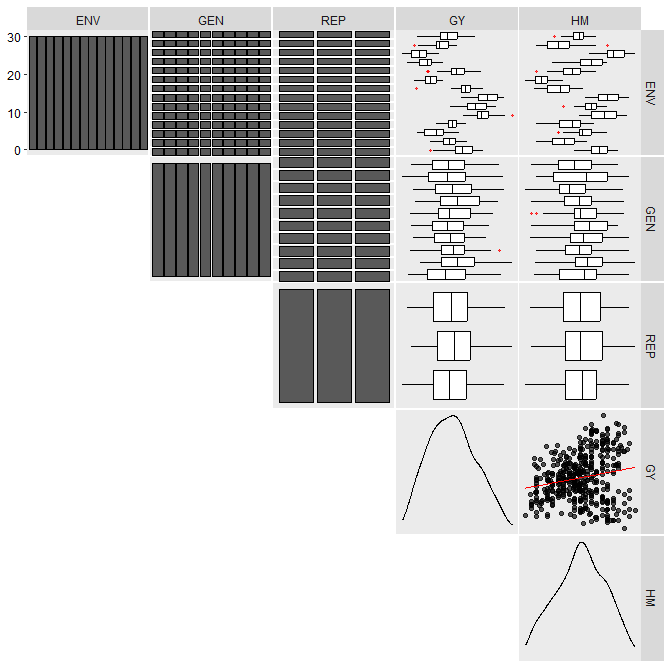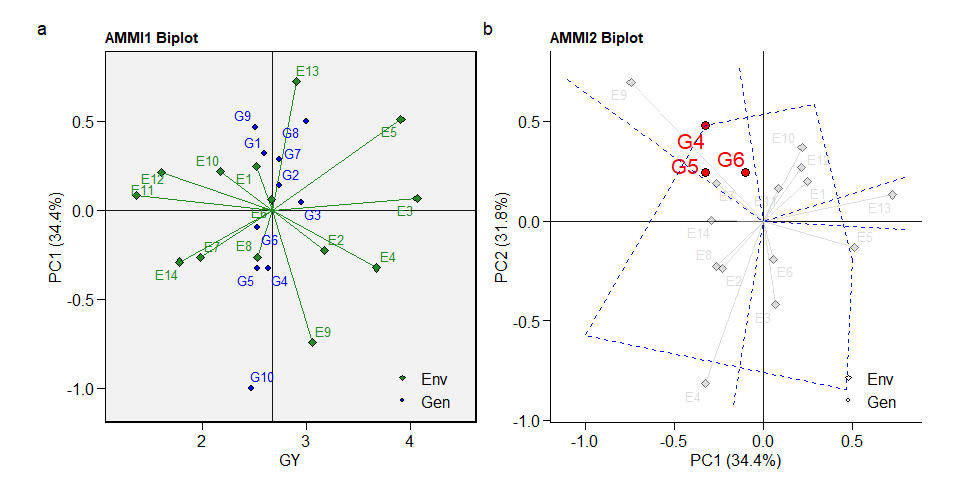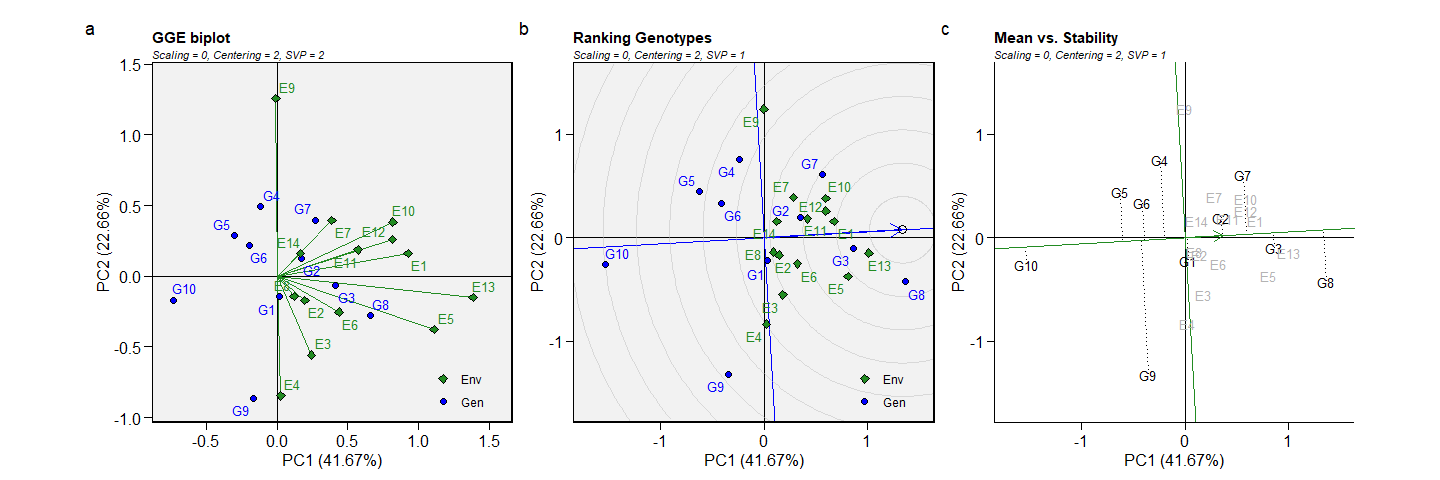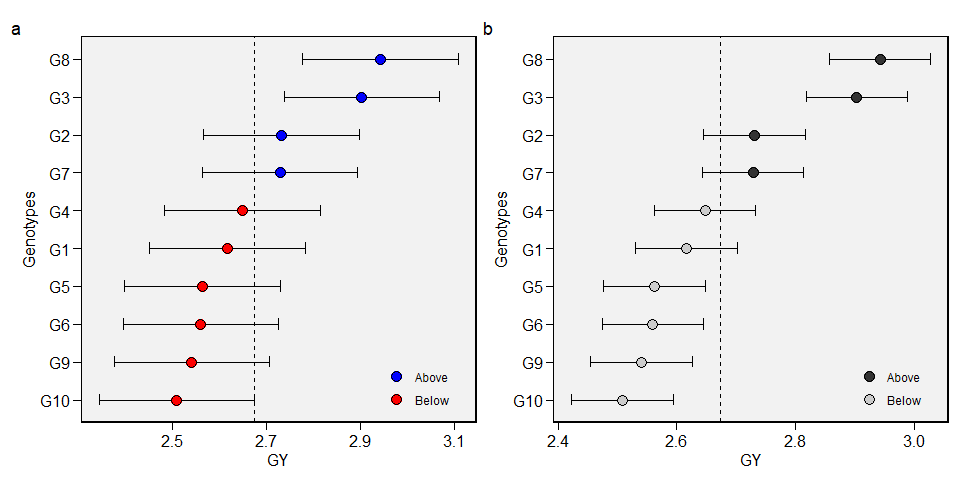metan (multi-environment trials analysis) provides useful functions for analyzing multi-environment trial data using parametric and nonparametric methods. The package will help you to:

• Inspect data for possible common errors;
• Manipulate rows and columns;
• Manipulate numbers and strings;
• Compute descriptive statistics;
• Compute within-environment analysis of variance;
• Compute AMMI analysis with prediction considering different numbers of interaction principal component axes;
• Compute AMMI-based stability indexes;
• Compute GGE biplot analysis;
• Compute BLUP-based stability indexes;
• Compute variance components and genetic parameters in mixed-effect models;
• Perform cross-validation procedures for AMMI-family and BLUP models;
• Compute parametric and nonparametric stability statistics
• Implement biometrical models

For more details see the complete vignette.

# Installation

Install the released version of metan from CRAN with:

install.packages("metan")

Or install the development version from GitHub with:

devtools::install_github("TiagoOlivoto/metan")

# To build the HTML vignette use
devtools::install_github("TiagoOlivoto/metan", build_vignettes = TRUE)

For the latest release notes on this development version, see the NEWS file.

# Getting started

metan offers a set of functions that can be used to manipulate, summarize, analyze and plot typical multi-environment trial data. Maybe, one of the first functions users should use would be inspect(). Here, we will inspect the example dataset data_ge that contains data on two variables assessed in 10 genotypes growing in 14 environments.

library(metan)

inspect(data_ge, plot = TRUE)
# # A tibble: 5 x 9
#   Variable Class   Missing Levels Valid_n   Min Median   Max Outlier
#   <chr>    <chr>   <chr>   <chr>    <int> <dbl>  <dbl> <dbl>   <dbl>
# 1 ENV      factor  No      14         420 NA     NA    NA         NA
# 2 GEN      factor  No      10         420 NA     NA    NA         NA
# 3 REP      factor  No      3          420 NA     NA    NA         NA
# 4 GY       numeric No      -          420  0.67   2.61  5.09       0
# 5 HM       numeric No      -          420 38     48    58          0No issues while inspecting the data. If any issue is given here (like outliers, missing values, etc.) consider using find_outliers() to find possible outliers in the data set or any metan’s data manipulation tool such as remove_na_rows() to remove rows with NA values, to_factor() to convert desired columns to factor, find_text_in_num() to find text fragments in columns assumed to be numeric, or even tidy_strings() to tidy up strings.

# Descriptive statistics

metan provides a set of functions to compute descriptive statistics. The easiest way to do that is by using desc_stat().

desc_stat(data_ge2)
# # A tibble: 15 x 9
#    variable    cv     max    mean  median     min  sd.amo     se      ci
#    <chr>    <dbl>   <dbl>   <dbl>   <dbl>   <dbl>   <dbl>  <dbl>   <dbl>
#  1 CD        7.34  18.6    16.0    16      12.9    1.17   0.0939  0.186
#  2 CDED      5.71   0.694   0.586   0.588   0.495  0.0334 0.0027  0.0053
#  3 CL        7.95  34.7    29.0    28.7    23.5    2.31   0.185   0.365
#  4 CW       25.2   38.5    24.8    24.5    11.1    6.26   0.501   0.99
#  5 ED        5.58  54.9    49.5    49.9    43.5    2.76   0.221   0.437
#  6 EH       21.2    1.88    1.34    1.41    0.752  0.284  0.0228  0.045
#  7 EL        8.28  17.9    15.2    15.1    11.5    1.26   0.101   0.199
#  8 EP       10.5    0.660   0.537   0.544   0.386  0.0564 0.0045  0.0089
#  9 KW       18.9  251.    173.    175.    106.    32.8    2.62    5.18
# 10 NKE      14.2  697.    512.    509.    332.    72.6    5.82   11.5
# 11 NKR      10.7   42      32.2    32      23.2    3.47   0.277   0.548
# 12 NR       10.2   21.2    16.1    16      12.4    1.64   0.131   0.259
# 13 PERK      2.17  91.8    87.4    87.5    81.2    1.90   0.152   0.300
# 14 PH       13.4    3.04    2.48    2.52    1.71   0.334  0.0267  0.0528
# 15 TKW      13.9  452.    339.    342.    218.    47.1    3.77    7.44

# AMMI model

## Fitting the model

The AMMI model is fitted with the function performs_ammi(). To analyze multiple variables at once we can use a comma-separated vector of unquoted variable names, or use any select helper in the argument resp. Here, using everything() we apply the function to all numeric variables in the data. For more details, see the complete vignette.

model <- performs_ammi(data_ge,
env = ENV,
gen = GEN,
rep = REP,
resp = everything(),
verbose = FALSE)
# Significance of IPCAs
get_model_data(model, "ipca_pval")
# Class of the model: performs_ammi
# Variable extracted: Pr(>F)
# # A tibble: 9 x 4
#   PC       DF      GY     HM
#   <chr> <dbl>   <dbl>  <dbl>
# 1 PC1      21 0       0
# 2 PC2      19 0       0
# 3 PC3      17 0.0014  0.0021
# 4 PC4      15 0.00960 0.0218
# 5 PC5      13 0.318   0.0377
# 6 PC6      11 0.561   0.041
# 7 PC7       9 0.754   0.0633
# 8 PC8       7 0.804   0.232
# 9 PC9       5 0.934   0.944

## Biplots

The well-known AMMI1 and AMMI2 biplots can be created with plot_scores(). Note that since performs_ammi allows analyzing multiple variables at once, e.g., resp = c(v1, v2, ...), the output model is a list, in this case with two elements (GY and HM). By default, the biplots are created for the first variable of the model. To choose another variable use the argument var (e.g., var = "HM").

a <- plot_scores(model)
b <- plot_scores(model,
type = 2,
polygon = TRUE,
col.env = "gray70",
col.segm.env = "gray70",
axis.expand = 1.5)
c <- plot_scores(model, type = 4)
arrange_ggplot(a, b, c, labels = letters[1:3], nrow = 1)# GGE model

The GGE model is fitted with the function gge(). For more details, see the complete vignette.

model <- gge(data_ge, ENV, GEN, GY)
model2 <- gge(data_ge, ENV, GEN, GY, svp = "genotype")
model3 <- gge(data_ge, ENV, GEN, GY, svp = "symmetrical")
d <- plot(model)
e <- plot(model2, type = 8)
f <- plot(model2,
type = 2,
col.gen = "black",
col.env = "gray70",
axis.expand = 1.5)
arrange_ggplot(d, e, f, labels = letters[4:6], nrow = 1)# BLUP model

Linear-mixed effect models to predict the response variable in METs are fitted using the function waasb(). Here we will obtain the predicted means for genotypes in the variables GY and HM. For more details, see the complete vignette.

model2 <- waasb(data_ge,
env = ENV,
gen = GEN,
rep = REP,
resp = everything())
# Method: REML/BLUP
# Random effects: GEN, GEN:ENV
# Fixed effects: ENV, REP(ENV)
# ---------------------------------------------------------------------------
# P-values for Likelihood Ratio Test of the analyzed traits
# ---------------------------------------------------------------------------
#     model       GY       HM
#  COMPLETE       NA       NA
#       GEN 1.11e-05 5.07e-03
#   GEN:ENV 2.15e-11 2.27e-15
# ---------------------------------------------------------------------------
# All variables with significant (p < 0.05) genotype-vs-environment interaction
# Get the variance components
get_model_data(model2, what = "vcomp")
# Class of the model: waasb
# Variable extracted: vcomp
# # A tibble: 3 x 3
#   Group        GY    HM
#   <chr>     <dbl> <dbl>
# 1 GEN      0.0280 0.490
# 2 GEN:ENV  0.0567 2.19
# 3 Residual 0.0967 2.84

## Plotting the BLUPs for genotypes

To produce a plot with the predicted means, use the function plot_blup().

g <- plot_blup(model2)
h <- plot_blup(model2,
prob = 0.1,
col.shape  =  c("gray20", "gray80")) + ggplot2::coord_flip()
arrange_ggplot(g, h, labels = letters[7:8])# Computing parametric and non-parametric stability indexes

The easiest way to compute parametric and non-parametric stability indexes in metan is by using the function ge_stats(). It is a wrapper function around a lot of specific functions for stability indexes. To get the results into a “ready-to-read” file, use get_model_data() or its shortcut gmd().

stats <- ge_stats(data_ge, ENV, GEN, REP, GY)
get_model_data(stats)
# Class of the model: ge_stats
# Variable extracted: stats
# # A tibble: 10 x 33
#    var   gen       Y    CV   Var Shukla  Wi_g  Wi_f  Wi_u Ecoval   bij      Sij
#    <chr> <chr> <dbl> <dbl> <dbl>  <dbl> <dbl> <dbl> <dbl>  <dbl> <dbl>    <dbl>
#  1 GY    G1     2.60  35.2 10.9  0.0280  84.4  89.2  81.1  1.22  1.06  -0.00142
#  2 GY    G10    2.47  42.3 14.2  0.244   59.2  64.6  54.4  7.96  1.12   0.177
#  3 GY    G2     2.74  34.0 11.3  0.0861  82.8  95.3  75.6  3.03  1.05   0.0497
#  4 GY    G3     2.96  29.9 10.1  0.0121 104.   99.7 107.   0.725 1.03  -0.0128
#  5 GY    G4     2.64  31.4  8.93 0.0640  85.9  79.5  91.9  2.34  0.937  0.0298
#  6 GY    G5     2.54  30.6  7.82 0.0480  82.7  82.2  82.4  1.84  0.887  0.00902
#  7 GY    G6     2.53  29.7  7.34 0.0468  83.0  83.7  81.8  1.81  0.861  0.00304
#  8 GY    G7     2.74  27.4  7.33 0.122   83.9  77.6  93.4  4.16  0.819  0.0579
#  9 GY    G8     3.00  30.4 10.8  0.0712  98.8  90.5 107.   2.57  1.03   0.0382
# 10 GY    G9     2.51  42.4 14.7  0.167   68.8  68.9  70.3  5.56  1.19   0.0938
# # ... with 21 more variables: R2 <dbl>, ASV <dbl>, SIPC <dbl>, EV <dbl>,
# #   ZA <dbl>, WAAS <dbl>, HMGV <dbl>, RPGV <dbl>, HMRPGV <dbl>, Pi_a <dbl>,
# #   Pi_f <dbl>, Pi_u <dbl>, Gai <dbl>, S1 <dbl>, S2 <dbl>, S3 <dbl>, S6 <dbl>,
# #   N1 <dbl>, N2 <dbl>, N3 <dbl>, N4 <dbl>

# Citation

citation("metan")

Olivoto, T., and Lúcio, A.D. (2020). metan: an R package for
multi-environment trial analysis. Methods Ecol Evol. Accepted Author
Manuscript doi:10.1111/2041-210X.13384

A BibTeX entry for LaTeX users is

@Article{Olivoto2020,
author = {Tiago Olivoto and Alessandro Dal'Col L{'{u}}cio},
title = {metan: an R package for multi-environment trial analysis},
journal = {Methods in Ecology and Evolution},
volume = {n/a},
number = {n/a},
year = {2020},
doi = {10.1111/2041-210X.13384},
url = {https://besjournals.onlinelibrary.wiley.com/doi/abs/10.1111/2041-210X.13384},
eprint = {https://besjournals.onlinelibrary.wiley.com/doi/pdf/10.1111/2041-210X.13384},
}

# Getting help

• If you encounter a clear bug, please file a minimal reproducible example on github

• Suggestions and criticisms to improve the quality and usability of the package are welcome!